# Faster R-CNN | ML

After the improvement in architecture of object detection network in R-CNN to Fast R_CNN. The training and detection time of the network decrease considerably, but the network is not fast enough to be used as a real-time system because it takes approximately (2 seconds) to generate output on an input image. The bottleneck of architecture is a selective search algorithm. Therefore K He et al. proposed a new architecture called Faster R-CNN. It does not use selective search instead they propose another region proposal generation algorithm called Region Proposal Network. Let’s discuss the Faster R-CNN architecture.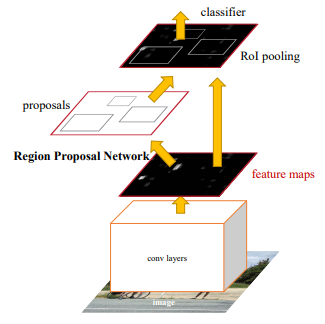Faster R-CNN architecture

Faster R-CNN architecture contains 2 networks:

1. Region Proposal Network (RPN)
2. Object Detection Network

Before discussing the Region proposal we need to look into the CNN architecture which is the backbone of this network. This CNN architecture is common between both Region Proposal Network and Object Detection Network. We experimented with ZF (which has 5 shareable Conv layers) or VGG-16 (which has 13 shareable Conv) as the backbone in their architecture. Both backbone network has the network stride of 16 which means an image of dimension 1000 * 600 is reduced to (1000/16 * 600/16) or approximately (~ 62 *37) size feature map before passing into region proposal network.

Region Proposal Network (RPN):
This region proposal network takes convolution feature map that is generated by the backbone layer as input and outputs the anchors generated by sliding window convolution applied on the input feature map.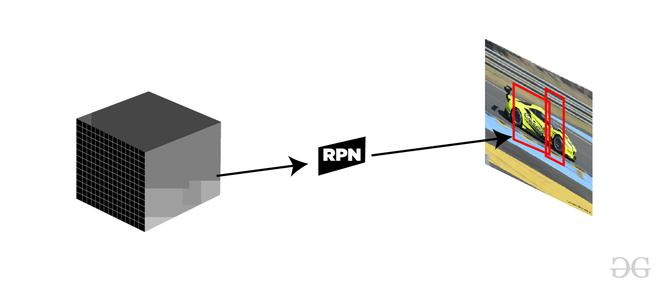Function of RPN (Feature Map to Region Proposal)

Anchors:
For each sliding window, the network generates the maximum number of k- anchor boxes. By the default the value of k=9 (3 scales of (128*128, 256*256 and 512*512) and 3 aspect ratio of (1:1, 1:2 and 2:1)) for each of different sliding position in image. Therefore, for a convolution feature map of W * H, we get N = W* H* k anchor boxes. These region proposals then passed into an intermediate layer of 3*3 convolution and 1 padding and 256 (for ZF) or 512 (for VGG-16 ) output channels. The output generated from this layer is passed into two layers of 1*1 convolution, the classification layer, and the regression layer. the regression layer has 4*N (W * H * (4*k)) output parameters (denoting the coordinates of bounding boxes) and the classification layer has 2*N (W * H * (2*k)) output parameters (denoting the probability of object or not object).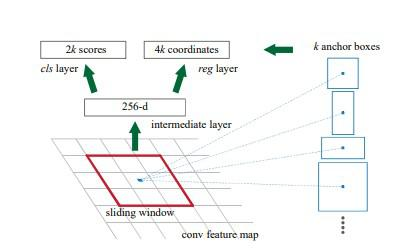Anchor generation

Training and Loss Function (RPN) :
First of all, we remove all the cross-boundary anchors, so, that they do not increase the loss function. For a typical 1000*600 image, there are roughly 20000(~ 60*40*9) anchors. If we remove the cross-boundary anchors then there are roughly 6000 anchors left per image. The paper also uses Non-Maximum Suppression based on their classification and IoU. Here they use a fixed IoU of 0.7. This also reduces the number of anchors to 2000. The advantage of using Non-Maximum suppression that it also doesn’t hurt accuracy as well. RPN can be trained end to end by using backpropagation and stochastic gradient descent. It generates each mini-batch from the anchors of a single image. It does not train loss function on each anchor instead it selects 256 random anchors with positive and negative sample s in the ratio of 1:1. If an image contains <128 positives then it uses more negative samples. For training RPNs, First, we need to assign binary class label (weather the concerned anchor contains an object or background). In the faster R-CNN paper, the author uses two conditions to assign a positive label to an anchor. These are :

• those anchors which have the highest Intersection-over-Union (IoU) with a ground-truth box, or
• an anchor that has an IoU overlap higher than 0.7 with any ground-truth box.

and negative label to those which has IoU overlap is <0.3 for all ground truth boxes. Those anchors which does not have either positive or negative label does not contribute to training. Now Loss function is defined as follows :where,
pi = predicted probability of anchors contains an object or not.
pi* = ground truth value of anchors contains and object or not.
ti = coordinates of predicted anchors.
ti* = ground truth coordinate associated with bounding boxes.
Lcls = Classifier Loss (binary log loss over two classes).
Lreg = Regression Loss (Here, Lreg  = R(ti-ti*) where R is smooth L1 loss)
Ncls = Normalization parameter of mini-batch size (~256).
Nreg = Normalization parameter of regression (equal to number of anchor locations ~2400).In order to make n=btoh loss parameter equally weighted right.

Object Detection Network:
The object detection network used in Faster R-CNN is very much similar to that used in Fast R-CNN. It is also compatible with VGG-16 as a backbone network. It also uses the RoI pooling layer for making region proposal of fixed size and twin layers of softmax classifier and the bounding box regressor is also used in the prediction of the object and its bounding box.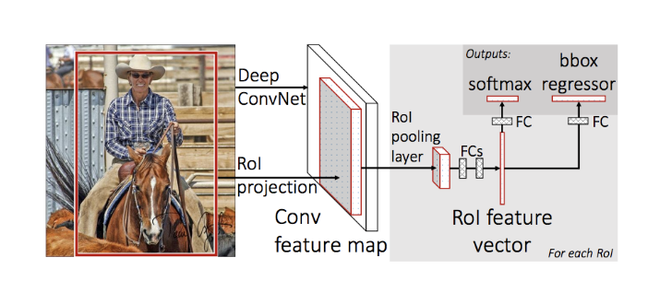Fast R-CNN architecture

RoI pooling :
We take the output generated from region proposal as input and passed into the RoI pooling layer, this RoI pooling layer has the same function as it performed in Fast R-CNN, to make different sizes region proposals generated from RPN into a fixed-size feature map. We have discussed RoI pooling in this article in great detail. This RoI pooling layer generates the output of size (7*7*D) (where D =256 for ZF and 512 of VGG-16).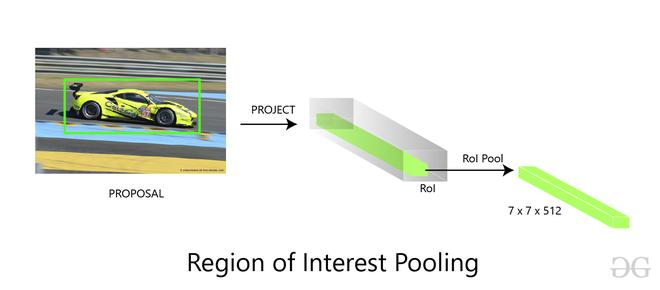Softmax and Bounding Box Regression Layer:
The feature map of size (7 * 7 * D) generated in RoI pooling are then sent to two fully connected layers, these fully connected layers flatten the feature maps and then send the output into two parallel fully connected layer each with the different task assigned to them:

• The first layer is a softmax layer of N+1 output parameters (N is the number of class labels and background ) that predicts the objects in the region proposal. The second layer is a bounding box regression layer that has 4* N output parameters. This layer regresses the bounding box location of the object in the image.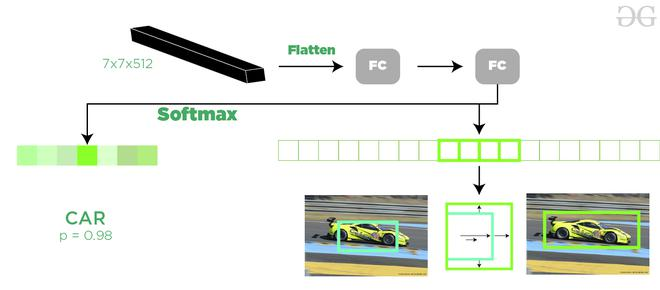Softmax Classifier and Bounding Box Regressor

Training (Full Architecture):
We have discussed training the RPN but in this part, we will discuss training the whole architecture. The authors of Faster R-CNN papers use an approach called 4 steps alternating training method. This approach is as follows

• We first initialize the backbone CNN network with ImageNet weights and fine-tuned these weights for region proposal. Now, we trained the RPN as described above.
• We separately trained the object detection network using the proposal generated by the RPN network. In this part also the backbone network is initialized with ImageNet weight and until now it is not connected to the RPN network.
• The RPN is now initialized with weights from a detector network (Fast R-CNN). This time only the weights of layers unique to the RPN are fine-tuned.
• Using the new fine-tuned RPN, the Fast R-CNN detector is fine-tuned. Again, only the layers unique to the detector network are fine-tuned and the common layer weights are fixed.

Results and Conclusion:

• Since the bottleneck of Fast R-CNN architecture is region proposal generation with the selective search. Faster R-CNN replaced it with its own Region Proposal Network. This Region proposal network is faster as compared to selective and it also improves region proposal generation model while training. This also helps us reduce the overall detection time as compared to fast R-CNN (0.2 seconds with Faster R-CNN (VGG-16 network) as compared to 2.3 in Fast R-CNN).
• Faster R-CNN (with RPN and VGG shared) when trained with COCO, VOC 2007 and VOC 2012 dataset generates mAP of 78.8% against 70% in Fast R-CNN on VOC 2007 test dataset)
• Region Proposal Network (RPN) when compared to selective search, also contributed marginally to the improvement of mAP.

References :

My Personal Notes arrow_drop_upCheck out this Author's contributed articles.

If you like GeeksforGeeks and would like to contribute, you can also write an article using contribute.geeksforgeeks.org or mail your article to contribute@geeksforgeeks.org. See your article appearing on the GeeksforGeeks main page and help other Geeks.

Please Improve this article if you find anything incorrect by clicking on the "Improve Article" button below.

Article Tags :
Practice Tags :

Be the First to upvote.

Please write to us at contribute@geeksforgeeks.org to report any issue with the above content.